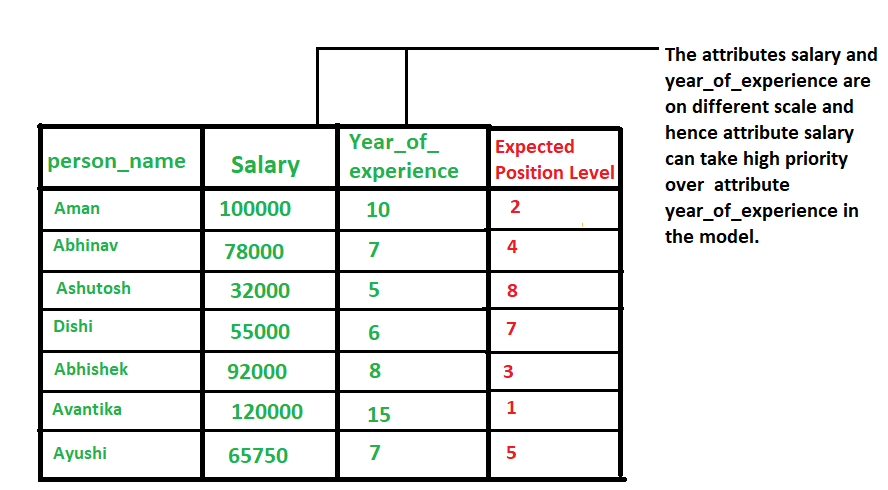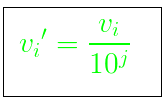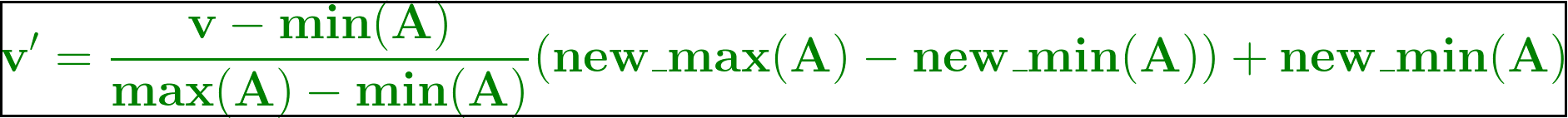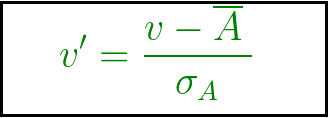GeeksforGeeks App
Open AppBrowser
Continue

# Data Normalization in Data Mining

### INTRODUCTION:

Data normalization is a technique used in data mining to transform the values of a dataset into a common scale. This is important because many machine learning algorithms are sensitive to the scale of the input features and can produce better results when the data is normalized.

### There are several different normalization techniques that can be used in data mining, including:

1. Min-Max normalization: This technique scales the values of a feature to a range between 0 and 1. This is done by subtracting the minimum value of the feature from each value, and then dividing by the range of the feature.
2. Z-score normalization: This technique scales the values of a feature to have a mean of 0 and a standard deviation of 1. This is done by subtracting the mean of the feature from each value, and then dividing by the standard deviation.
3. Decimal Scaling: This technique scales the values of a feature by dividing the values of a feature by a power of 10.
4. Logarithmic transformation: This technique applies a logarithmic transformation to the values of a feature. This can be useful for data with a wide range of values, as it can help to reduce the impact of outliers.
5. Root transformation: This technique applies a square root transformation to the values of a feature. This can be useful for data with a wide range of values, as it can help to reduce the impact of outliers.
6. It’s important to note that normalization should be applied only to the input features, not the target variable, and that different normalization technique may work better for different types of data and models.

In conclusion, normalization is an important step in data mining, as it can help to improve the performance of machine learning algorithms by scaling the input features to a common scale. This can help to reduce the impact of outliers and improve the accuracy of the model.

Normalization is used to scale the data of an attribute so that it falls in a smaller range, such as -1.0 to 1.0 or 0.0 to 1.0. It is generally useful for classification algorithms.

### Need of Normalization –

Normalization is generally required when we are dealing with attributes on a different scale, otherwise, it may lead to a dilution in effectiveness of an important equally important attribute(on lower scale) because of other attribute having values on larger scale. In simple words, when multiple attributes are there but attributes have values on different scales, this may lead to poor data models while performing data mining operations. So they are normalized to bring all the attributes on the same scale.Methods of Data Normalization –

• Decimal Scaling
• Min-Max Normalization
• z-Score Normalization(zero-mean Normalization)

### Decimal Scaling Method For Normalization –

It normalizes by moving the decimal point of values of the data. To normalize the data by this technique, we divide each value of the data by the maximum absolute value of data. The data value, vi, of data is normalized to vi by using the formula below –where j is the smallest integer such that max(|vi‘|)<1. Example –

Let the input data is: -10, 201, 301, -401, 501, 601, 701 To normalize the above data, Step 1: Maximum absolute value in given data(m): 701 Step 2: Divide the given data by 1000 (i.e j=3) Result: The normalized data is: -0.01, 0.201, 0.301, -0.401, 0.501, 0.601, 0.701

### Min-Max Normalization –

In this technique of data normalization, linear transformation is performed on the original data. Minimum and maximum value from data is fetched and each value is replaced according to the following formula.Where A is the attribute data, Min(A), Max(A) are the minimum and maximum absolute value of A respectively. v’ is the new value of each entry in data. v is the old value of each entry in data. new_max(A), new_min(A) is the max and min value of the range(i.e boundary value of range required) respectively.

### Z-score normalization –

In this technique, values are normalized based on mean and standard deviation of the data A. The formula used is:v’, v is the new and old of each entry in data respectively. σA, A is the standard deviation and mean of A respectively.

Data normalization in data mining can have a number of advantages and disadvantages.

1. Improved performance of machine learning algorithms: Normalization can help to improve the performance of machine learning algorithms by scaling the input features to a common scale. This can help to reduce the impact of outliers and improve the accuracy of the model.
2. Better handling of outliers: Normalization can help to reduce the impact of outliers by scaling the data to a common scale, which can make the outliers less influential.
3. Improved interpretability of results: Normalization can make it easier to interpret the results of a machine learning model, as the inputs will be on a common scale.
4. Better generalization: Normalization can help to improve the generalization of a model, by reducing the impact of outliers and by making the model less sensitive to the scale of the inputs.